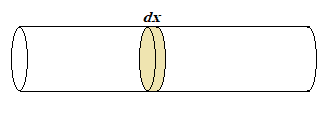# Calculating Linear Charge Density of a Cylinder

• chichiba
Yes. Substituting in the expression for ##\lambda## and noting that ##\rho(r) = -\frac{1}{2} \rho_{0}(r)##:dQ = -\frac{1}{2} \rho_{0}(r) \left( 1 - \frac{1}{2} \rho_{0}(r) \right)f

#### chichiba

Homework Statement
An infinitely long cylinder carries a charge density throughout its volume given by:
𝜌(𝑟) = 𝜌0 (𝛼 − 𝑟 /𝛽)
where 𝑟 is the radial distance from its central axis and 𝜌0, 𝛼, and 𝛽 are positive constants. The radius of the cylinder is 𝑅 beyond which the charge density is zero.
(a) Obtain an expression for the linear charge density of the cylinder.
(b) Obtain expressions for the electric field inside (𝑟 < 𝑅) and outside (𝑟 > 𝑅) the cylinder.
(c) Taking 𝑉(𝑅) = 0 as the reference, get the potential of the cylinder for points inside and outside the cylinder.
Relevant Equations
Linear charge density, Gauss's Law
For part a:

I know that linear charge density is the amount of charge per unit length, and we are given the volume charge density. Since we are given the volume, we can obtain the length by multiplying the volume by the cross sectional area, so C/m^3 * m^2 = C/m. The cross sectional area of a cylinder is A= πR^2, so we can get the linear charge density by solving for λ=𝜌A, and multiply our area by our initial equation to get:

λ = πR^2 𝜌0 (𝛼 − 𝑟/𝛽)

I haven't began part b or c yet, because I am not confident in part a. I do think that in both cases, for r>R (outside of the cylinder), that the electric field and potential would be zero, because R is beyond where the charge density is zero.

I also know to use Gauss's Law for part b, and integration for part c, but am not entirely sure where to begin.

Thank you!

Homework Statement:: An infinitely long cylinder carries a charge density throughout its volume given by:
𝜌(𝑟) = 𝜌0 (𝛼 − 𝑟 /𝛽)
where 𝑟 is the radial distance from its central axis and 𝜌0, 𝛼, and 𝛽 are positive constants. The radius of the cylinder is 𝑅 beyond which the charge density is zero.
(a) Obtain an expression for the linear charge density of the cylinder.
(b) Obtain expressions for the electric field inside (𝑟 < 𝑅) and outside (𝑟 > 𝑅) the cylinder.
(c) Taking 𝑉(𝑅) = 0 as the reference, get the potential of the cylinder for points inside and outside the cylinder.
Relevant Equations:: Linear charge density, Gauss's Law

For part a:

I know that linear charge density is the amount of charge per unit length, and we are given the volume charge density. Since we are given the volume, we can obtain the length by multiplying the volume by the cross sectional area, so C/m^3 * m^2 = C/m. The cross sectional area of a cylinder is A= πR^2, so we can get the linear charge density by solving for λ=𝜌A, and multiply our area by our initial equation to get:

λ = πR^2 𝜌0 (𝛼 − 𝑟/𝛽)
Your method for finding ##\lambda## will only work if ##\rho## is uniform across a cross section of the cylinder.

Consider a section of the cylinder of infinitesimal length ##dx## and let ##dQ## be the total charge contained within this section. ##\lambda = dQ/dx##.Can you use ##\rho(r)## to find an expression for ##dQ##?